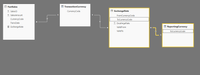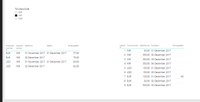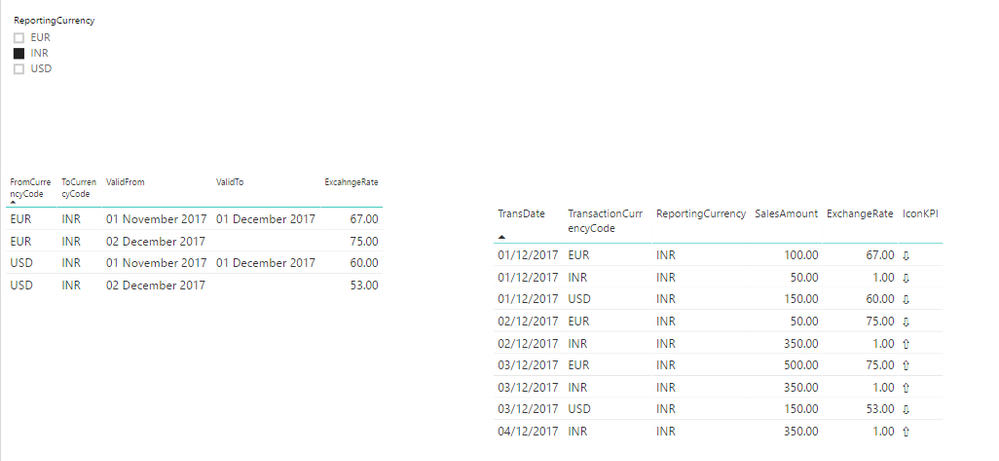cancel
Showing results for
Did you mean:Resolver I

## Dynamic Exchange Rate architecture and DAX calculation in Power BI

I want to get my amount to change as per currency code selected dynamically.

In my FactSales table I have currency and Amount. If user want to see that amount in any currency he select the currency from slicer and my excahnge rate will apply to that amount.

I tried below DAX to get dynamic Exchange Rate but no luck:

`ExchangeRate = CALCULATE(MIN(ExchangeRate[ExcahngeRate]), FILTER(ExchangeRate, AND(AND(AND(MIN(FactSales[TransDate])>=MIN(ExchangeRate[ValidFrom]), MIN(FactSales[TransDate])<=IF(ISBLANK(MIN(ExchangeRate[ValidTo])), DATEVALUE("01-01-2070"), MIN(ExchangeRate[ValidTo]))), MIN(ExchangeRate[FromCurrencyCode]) = MIN(FactSales[CurrencyCode])), MIN(ExchangeRate[ToCurrencyCode]) = FIRSTNONBLANK(ExchangeRate[ToCurrencyCode],1))))`

ExchangeRate = CALCULATE(MIN(ExchangeRate[ExcahngeRate]), FILTER(ExchangeRate, AND(AND(AND(MIN(FactSales[TransDate])>=MIN(ExchangeRate[ValidFrom]), MIN(FactSales[TransDate])<=IF(ISBLANK(MIN(ExchangeRate[ValidTo])), DATEVALUE("01-01-2070"), MIN(ExchangeRate[ValidTo]))), MIN(ExchangeRate[FromCurrencyCode]) = MIN(FactSales[CurrencyCode])), MIN(ExchangeRate[ToCurrencyCode]) = FIRSTNONBLANK(ExchangeRate[ToCurrencyCode],1))))1 ACCEPTED SOLUTIONResolver I

If we have Exchange rate on daily basis then we can go for below solution:

https://www.kasperonbi.com/currency-conversion-in-dax-for-power-bi-and-ssas/If we have Exchange Rate as date range then use dynamic DAX:

```ExchangeRate =
IF(ISBLANK
(
CALCULATE(MIN(ExchangeRate[ExcahngeRate]),
FILTER(FILTER(ExchangeRate, AND(AND(ExchangeRate[FromCurrencyCode] = MIN(FactSales[TransactionCurrencyCode]), ExchangeRate[ToCurrencyCode] = FIRSTNONBLANK(ExchangeRate[ToCurrencyCode],1))
, MIN(FactSales[TransDate])<=IF(ISBLANK(ExchangeRate[ValidTo].[Date]), DATEVALUE("2070-01-01"), ExchangeRate[ValidTo]))), MIN(FactSales[TransDate])>= ExchangeRate[ValidFrom]))
)
, 1
, CALCULATE(MIN(ExchangeRate[ExcahngeRate]),
FILTER(FILTER(ExchangeRate, AND(AND(ExchangeRate[FromCurrencyCode] = MIN(FactSales[TransactionCurrencyCode])
, ExchangeRate[ToCurrencyCode] = FIRSTNONBLANK(ExchangeRate[ToCurrencyCode],1))
, MIN(FactSales[TransDate])<=IF(ISBLANK(ExchangeRate[ValidTo].[Date]), DATEVALUE("2070-01-01"), ExchangeRate[ValidTo])))
, MIN(FactSales[TransDate])>= ExchangeRate[ValidFrom]))
)```Resolver I

If we have Exchange rate on daily basis then we can go for below solution:

https://www.kasperonbi.com/currency-conversion-in-dax-for-power-bi-and-ssas/If we have Exchange Rate as date range then use dynamic DAX:

```ExchangeRate =
IF(ISBLANK
(
CALCULATE(MIN(ExchangeRate[ExcahngeRate]),
FILTER(FILTER(ExchangeRate, AND(AND(ExchangeRate[FromCurrencyCode] = MIN(FactSales[TransactionCurrencyCode]), ExchangeRate[ToCurrencyCode] = FIRSTNONBLANK(ExchangeRate[ToCurrencyCode],1))
, MIN(FactSales[TransDate])<=IF(ISBLANK(ExchangeRate[ValidTo].[Date]), DATEVALUE("2070-01-01"), ExchangeRate[ValidTo]))), MIN(FactSales[TransDate])>= ExchangeRate[ValidFrom]))
)
, 1
, CALCULATE(MIN(ExchangeRate[ExcahngeRate]),
FILTER(FILTER(ExchangeRate, AND(AND(ExchangeRate[FromCurrencyCode] = MIN(FactSales[TransactionCurrencyCode])
, ExchangeRate[ToCurrencyCode] = FIRSTNONBLANK(ExchangeRate[ToCurrencyCode],1))
, MIN(FactSales[TransDate])<=IF(ISBLANK(ExchangeRate[ValidTo].[Date]), DATEVALUE("2070-01-01"), ExchangeRate[ValidTo])))
, MIN(FactSales[TransDate])>= ExchangeRate[ValidFrom]))
)```